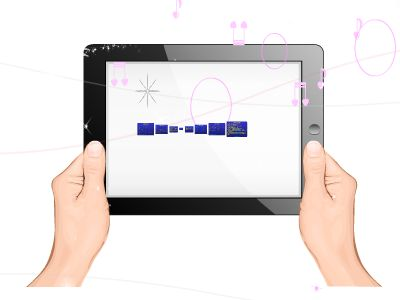Publications: 64 | Followers: 0

## toc03-28

Publish on Category: Birds 0

March 28, 2019
Theory of Computation Lecture 12: A Universal Program IV
1
Universality
Then we append the following instruction:[C] IF K = Lt(Z) + 1  K = 0 GOTO FSo if the computation has ended, GOTO F, where the proper value will be output.Otherwise, the current instruction is decoded and executed:U  r((Z)K)P  pr(U)+1Remember that (Z)K= a, b, c is the number of the K-th instruction.So U = b, c is the code of the statement to be executed.The variable mentioned in this statement is the (c + 1)-th, i.e., the (r(U) + 1)-th.
March 28, 2019
Theory of Computation Lecture 12: A Universal Program IV
2
Universality
Then l(U) contains the type of the instruction to be executed.IF l(U) = 0 GOTO NIF l(U) = 1 GOTO AIF (P | S) GOTO NIF l(U) = 2 GOTO MIf either the instruction is V  V, or the instruction is V  V-1 and V = 0 (as indicated by the absence of P in S), or the instruction is IF V0 GOTO L and V = 0, then nothing is done to S (“nothing” - GOTO N).If the instruction is V  V+1, then the exponent of P in S needs to be incremented (“add” – GOTO A).If the instruction is V  V-1 with V > 0, then the exponent of P in S needs to be decremented (“minus” – GOTO M).
March 28, 2019
Theory of Computation Lecture 12: A Universal Program IV
3
Universality
If none of the four previous predicates were true, a GOTO command has to be executed:K  miniLt(Z)[l((Z)i) + 2 = l(U)]GOTO CSo if the label l(U) – 2 exists in the program, the number K of the next instruction to be executed will be set to the first instruction with that label.Otherwise, K will be set to 0.As you remember, if K = 0 or K = Lt(Z) + 1, then the computation stops.In any case, our interpreter program executes a GOTO C to execute the next instruction.
March 28, 2019
Theory of Computation Lecture 12: A Universal Program IV
4
Universality
The program continues as follows:[M] S  S/PGOTO N[A] S  S  P[N] K  K+1GOTO CThe value of the variable in the current instruction is decremented or incremented by 1 by dividing or multiplying S by P, respectively.Then K is incremented and the next instruction executed.
March 28, 2019
Theory of Computation Lecture 12: A Universal Program IV
5
Universality
The program concludes with the following line:[F] Y  (S)1This way, after termination of the interpreted program, its output value becomes the output value of the interpreter.On the next slide, we will list the entire interpreter program.
March 28, 2019
Theory of Computation Lecture 12: A Universal Program IV
6
Universality
Z  Xn+1+ 1S  ni=1(p2i)XiK  1[C] IF K = Lt(Z) + 1 K = 0 GOTO FU  r((Z)K)P  pr(U)+1IF l(U) = 0 GOTO NIF l(U) = 1 GOTO AIF (P | S) GOTO NIF l(U) = 2 GOTO M
K  miniLt(Z)[l((Z)i) + 2 = l(U)]GOTO C[M] S  S/PGOTO N[A] S  S  P[N] K  K+1GOTO C[F] Y  (S)1
March 28, 2019
Theory of Computation Lecture 12: A Universal Program IV
7
Universality
For each n > 0, the sequence(n)(x1, …, xn, 0), (n)(x1, …, xn, 1), …enumerates all partially computable functions of n variables. We can also write:y(n)(x1, …, xn) = (n)(x1, …, xn, y).We can omit the superscript (n) when n = 1:y(x) = (x, y) = (1)(x, y).
March 28, 2019
Theory of Computation Lecture 12: A Universal Program IV
8
Universality
Consider the following predicates:STP(n)(x1, …,xn, y, t) Program number y halts after t or fewersteps on inputs x1, …,xn There is a computation of program y oflength  t + 1, beginning with inputs x1, …,xnThese predicates arecomputable, which we can easily prove:We can simply add acounterto our universal programs to determine when we have simulated t steps.

0

Embed

Share